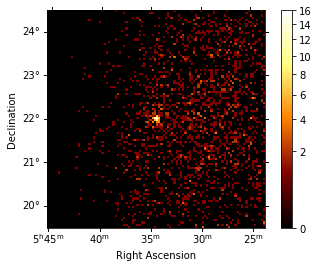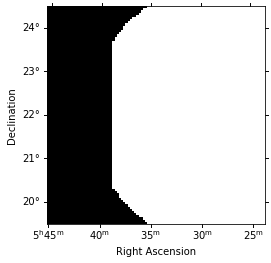This is a fixed-text formatted version of a Jupyter notebook

# Makers - Data reduction¶

## Introduction¶

The gammapy.makers sub-package contains classes to perform data reduction tasks from DL3 data to binned datasets. In the data reduction step the DL3 data is prepared for modeling and fitting, by binning events into a counts map and interpolating the exposure, background, psf and energy dispersion on the chosen analysis geometry.

## Setup¶

:

from gammapy.makers import (
MapDatasetMaker,
FoVBackgroundMaker,
SpectrumDatasetMaker,
ReflectedRegionsBackgroundMaker,
)
from gammapy.datasets import MapDataset, SpectrumDataset, Datasets
from gammapy.data import DataStore
from gammapy.maps import MapAxis, WcsGeom, RegionGeom
from regions import CircleSkyRegion
from astropy import units as u
import numpy as np


## Dataset¶

The counts, exposure, background and IRF maps are bundled together in a data structure named MapDataset. To handle on-off observations Gammapy also features a MapDatasetOnOff class, which stores in addition the counts_off, acceptance and acceptance_off data.

The first step of the data reduction is to create an empty dataset. A MapDataset can be created from any WcsGeom object. This is illustrated in the following example:

:

energy_axis = MapAxis.from_bounds(
1, 10, nbin=11, name="energy", unit="TeV", interp="log"
)
geom = WcsGeom.create(
skydir=(83.63, 22.01),
axes=[energy_axis],
width=5 * u.deg,
binsz=0.05 * u.deg,
frame="icrs",
)
dataset_empty = MapDataset.create(geom=geom)
print(dataset_empty)

MapDataset
----------

Name                            : FrY21vkA

Total counts                    : 0
Total background counts         : 0.00
Total excess counts             : 0.00

Predicted counts                : 0.00
Predicted background counts     : 0.00
Predicted excess counts         : nan

Exposure min                    : 0.00e+00 m2 s
Exposure max                    : 0.00e+00 m2 s

Number of total bins            : 110000
Number of fit bins              : 0

Fit statistic type              : cash
Fit statistic value (-2 log(L)) : nan

Number of models                : 0
Number of parameters            : 0
Number of free parameters       : 0



It is possible to compute the instrument response functions with different spatial and energy binnings as compared to the counts and background maps. For example, one can specify a true energy axis which defines the energy binning of the IRFs:

:

energy_axis_true = MapAxis.from_bounds(
0.3, 10, nbin=31, name="energy_true", unit="TeV", interp="log"
)
dataset_empty = MapDataset.create(geom=geom, energy_axis_true=energy_axis_true)


For the detail of the other options availables, you can always call the help:

:

help(MapDataset.create)

Help on method create in module gammapy.datasets.map:

create(geom, energy_axis_true=None, migra_axis=None, rad_axis=None, binsz_irf=None, reference_time='2000-01-01', name=None, meta_table=None, **kwargs) method of abc.ABCMeta instance
Create a MapDataset object with zero filled maps.

Parameters
----------
geom : ~gammapy.maps.WcsGeom
Reference target geometry in reco energy, used for counts and background maps
energy_axis_true : ~gammapy.maps.MapAxis
True energy axis used for IRF maps
migra_axis : ~gammapy.maps.MapAxis
If set, this provides the migration axis for the energy dispersion map.
If not set, an EDispKernelMap is produced instead. Default is None
rad_axis : ~gammapy.maps.MapAxis
Rad axis for the psf map
binsz_irf : float
IRF Map pixel size in degrees.
reference_time : ~astropy.time.Time
the reference time to use in GTI definition
name : str
Name of the returned dataset.
meta_table : ~astropy.table.Table
Table listing information on observations used to create the dataset.
One line per observation for stacked datasets.

Returns
-------
empty_maps : MapDataset
A MapDataset containing zero filled maps



Once this empty “reference” dataset is defined, it can be filled with observational data using the MapDatasetMaker:

:

# get observation
data_store = DataStore.from_dir("$GAMMAPY_DATA/hess-dl3-dr1") obs = data_store.get_observations() # fill dataset maker = MapDatasetMaker() dataset = maker.run(dataset_empty, obs) print(dataset) dataset.counts.sum_over_axes().plot(stretch="sqrt", add_cbar=True);  No HDU found matching: OBS_ID = 23592, HDU_TYPE = rad_max, HDU_CLASS = None  MapDataset ---------- Name : YQkm5bSN Total counts : 2016 Total background counts : 1866.72 Total excess counts : 149.28 Predicted counts : 1866.72 Predicted background counts : 1866.72 Predicted excess counts : nan Exposure min : 1.19e+02 m2 s Exposure max : 1.09e+09 m2 s Number of total bins : 110000 Number of fit bins : 110000 Fit statistic type : cash Fit statistic value (-2 log(L)) : nan Number of models : 0 Number of parameters : 0 Number of free parameters : 0The MapDatasetMaker fills the corresponding counts, exposure, background, psf and edisp map per observation. The MapDatasetMaker has a selection parameter, in case some of the maps should not be computed. There is also a background_oversampling parameter that defines the oversampling factor in energy used to compute the bakcground (default is None). ## Safe data range handling¶ To exclude the data range from a MapDataset, that is associated with high systematics on instrument response functions, a mask_safe can be defined. The mask_safe is a Map object with bool data type, which indicates for each pixel, whether it should be included in the analysis. The convention is that a value of True or 1 includes the pixel, while a value of False or 0 excludes a pixels from the analysis. To compute safe data range masks according to certain criteria, Gammapy provides a SafeMaskMaker class. The different criteria are given by the methodsargument, available options are : • aeff-default, uses the energy ranged specified in the DL3 data files, if available. • aeff-max, the lower energy threshold is determined such as the effective area is above a given percentage of its maximum • edisp-bias, the lower energy threshold is determined such as the energy bias is below a given percentage • offset-max, the data beyond a given offset radius from the observation center are excluded • bkg-peak, the energy threshold is defined as the upper edge of the energy bin with the highest predicted background rate. This method was introduced in the HESS DL3 validation paper: https://arxiv.org/pdf/1910.08088.pdf Multiple methods can be combined. Here is an example : :  safe_mask_maker = SafeMaskMaker( methods=["aeff-default", "offset-max"], offset_max="3 deg" ) dataset = maker.run(dataset_empty, obs) dataset = safe_mask_maker.run(dataset, obs) print(dataset.mask_safe) dataset.mask_safe.sum_over_axes().plot();  WcsNDMap geom : WcsGeom axes : ['lon', 'lat', 'energy'] shape : (100, 100, 11) ndim : 3 unit : dtype : boolThe SafeMaskMaker does not modify any data, but only defines the MapDataset.mask_safe attribute. This means that the safe data range can be defined and modified in between the data reduction and stacking and fitting. For a joint-likelihood analysis of multiple observations the safe mask is applied to the counts and predicted number of counts map during fitting. This correctly accounts for contributions (spill-over) by the PSF from outside the field of view. ## Background estimation¶ The background computed by the MapDatasetMaker gives the number of counts predicted by the background IRF of the observation. Because its actual normalization, or even its spectral shape, might be poorly constrained, it is necessary to correct it with the data themselves. This is the role of background estimation Makers. ### FoV background¶ If the background energy dependent morphology is well reproduced by the background model stored in the IRF, it might be that its normalization is incorrect and that some spectral corrections are necessary. This is made possible thanks to the gammapy.makers.FoVBackgroundMaker. This technique is recommended in most 3D data reductions. For more details and usage, see fov_background. Here we are going to use a gammapy.makers.FoVBackgroundMaker that will rescale the background model to the data excluding the region where a known source is present. For more details on the way to create exclusion masks see the mask maps notebook. :  circle = CircleSkyRegion(center=geom.center_skydir, radius=0.2 * u.deg) exclusion_mask = geom.region_mask([circle], inside=False) fov_bkg_maker = FoVBackgroundMaker( method="scale", exclusion_mask=exclusion_mask ) dataset = fov_bkg_maker.run(dataset)  Other backgrounds production methods are available as listed below. ### Ring background¶ If the background model does not reproduce well the morphology, a classical approach consists in applying local corrections by smoothing the data with a ring kernel. This allows to build a set of OFF counts taking into account the inperfect knowledge of the background. This is implemented in the gammapy.makers.RingBackgroundMaker which transforms the Dataset in a MapDatasetOnOff. This technique is mostly used for imaging, and should not be applied for 3D modeling and fitting. For more details and usage, see ring_background. ### Reflected regions background¶ In the absence of a solid background model, a classical technique in Cherenkov astronomy for 1D spectral analysis is to estimate the background in a number of OFF regions. When the background can be safely estimated as radially symmetric w.r.t. the pointing direction, one can apply the reflected regions background technique. This is implemented in the gammapy.makers.ReflectedRegionsBackgroundMaker which transforms a SpectrumDataset in a SpectrumDatasetOnOff. This technique is only used for 1D spectral analysis. For more details and usage, see reflected_background. ## Data reduction loop¶ The data reduction steps can be combined in a single loop to run a full data reduction chain. For this the MapDatasetMaker is run first and the output dataset is the passed on to the next maker step. Finally the dataset per observation is stacked into a larger map. :  data_store = DataStore.from_dir("$GAMMAPY_DATA/hess-dl3-dr1")
observations = data_store.get_observations([23523, 23592, 23526, 23559])

energy_axis = MapAxis.from_bounds(
1, 10, nbin=11, name="energy", unit="TeV", interp="log"
)
geom = WcsGeom.create(
skydir=(83.63, 22.01), axes=[energy_axis], width=5, binsz=0.02
)

maker = MapDatasetMaker()
methods=["aeff-default", "offset-max"], offset_max="3 deg"
)

stacked = MapDataset.create(geom)

for obs in observations:
dataset = maker.run(cutout, obs)
dataset = fov_bkg_maker.run(dataset)
stacked.stack(dataset)

print(stacked)

No HDU found matching: OBS_ID = 23523, HDU_TYPE = rad_max, HDU_CLASS = None
No HDU found matching: OBS_ID = 23592, HDU_TYPE = rad_max, HDU_CLASS = None
No HDU found matching: OBS_ID = 23526, HDU_TYPE = rad_max, HDU_CLASS = None
No HDU found matching: OBS_ID = 23559, HDU_TYPE = rad_max, HDU_CLASS = None

MapDataset
----------

Name                            : jEMb_Ng9

Total counts                    : 7972
Total background counts         : 7555.42
Total excess counts             : 416.58

Predicted counts                : 7555.42
Predicted background counts     : 7555.42
Predicted excess counts         : nan

Exposure min                    : 1.04e+06 m2 s
Exposure max                    : 3.22e+09 m2 s

Number of total bins            : 687500
Number of fit bins              : 687214

Fit statistic type              : cash
Fit statistic value (-2 log(L)) : nan

Number of models                : 0
Number of parameters            : 0
Number of free parameters       : 0



To maintain good performance it is always recommended to do a cutout of the MapDataset as shown above. In case you want to increase the offset-cut later, you can also choose a larger width of the cutout than 2 * offset_max.

Note that we stack the individual MapDataset, which are computed per observation into a larger dataset. During the stacking the safe data range mask (MapDataset.mask_safe) is applied by setting data outside to zero, then data is added to the larger map dataset. To stack multiple observations, the larger dataset must be created first.

## Spectrum dataset¶

The spectrum datasets represent 1D spectra along an energy axis whitin a given on region. The SpectrumDataset contains a counts spectrum, and a background model. The SpectrumDatasetOnOff contains ON and OFF count spectra, background is implicitly modeled via the OFF counts spectrum.

The SpectrumDatasetMaker make spectrum dataset for a single observation. In that case the irfs and background are computed at a single fixed offset, which is recommend only for point-sources.

Here is an example of data reduction loop to create SpectrumDatasetOnOff datasets:

:

# on region is given by the CircleSkyRegion previously defined
geom = RegionGeom.create(region=circle, axes=[energy_axis])
np.logical_or, keepdims=False
)

spectrum_dataset_empty = SpectrumDataset.create(
geom=geom, energy_axis_true=energy_axis_true
)

spectrum_dataset_maker = SpectrumDatasetMaker(
containment_correction=False, selection=["counts", "exposure", "edisp"]
)
reflected_bkg_maker = ReflectedRegionsBackgroundMaker(
)

datasets = Datasets()

for observation in observations:
dataset = spectrum_dataset_maker.run(
spectrum_dataset_empty.copy(name=f"obs-{observation.obs_id}"),
observation,
)
dataset_on_off = reflected_bkg_maker.run(dataset, observation)
datasets.append(dataset_on_off)
print(datasets)

Datasets
--------

Dataset 0:

Type       : SpectrumDatasetOnOff
Name       : obs-23523
Instrument : HESS
Models     :

Dataset 1:

Type       : SpectrumDatasetOnOff
Name       : obs-23592
Instrument : HESS
Models     :

Dataset 2:

Type       : SpectrumDatasetOnOff
Name       : obs-23526
Instrument : HESS
Models     :

Dataset 3:

Type       : SpectrumDatasetOnOff
Name       : obs-23559
Instrument : HESS
Models     :


[ ]: